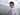# Haskell小白之 区分 (\$) 和 (.) 两个函数

June 03, 2017

``````(\$) :: (a -> b) -> a -> b
(.) :: (b -> c) -> (a -> b) -> a -> c``````

## (\$)

``f \$ x``

``````f a b c
(((f a) b) c)
-- 但是如果你的需求是这样的呢
f a (b c)
-- 即先计算最右侧的表达式的值，那我们就可以用 \$ 来代替括号了
f a \$ b c``````

``````3 * (1 + 2)
-- 换成用 \$ 表达 可以写成
(*) 3 \$ 1 + 2``````

``````sum (filter (>10) (map (*2) [2..10]))
-- 将2-10这9个数字分别乘2得到一个新数组，然后过滤掉数组中大于10的数，将剩余的
-- 数字求和，最后结果为80
-- 使用 \$ 后，变成了这样
sum \$ filter (>10) \$ map (*2) [2..10]

sum (map sqrt [1..130])
-- 等价于
sum \$ map sqrt [1..130]

length (takeWhile (<1000) (scanl1 (+) (map sqrt [1..]))) + 1
-- 等价于
(+1) \$ length \$ takeWhile (<1000) \$ scanl1 (+) \$ map sqrt [1..]``````

## (.)

``````-- 数学表述如下：
-- f(x) = x + 1
-- g(x) = 2x
-- o(x) = f(g(x))
-- 现在我们要的到o这样一个函数，使用函数组合就是下面这个样子
(+1).(*2)
-- 等价于
(\x -> x * 2) + 1``````

(.) 和 (\$) 配合起来使用，可以省去很多括号，让代码看起来十分简洁(前方高能):

``````-- 不使用的写法
replicate 2 (product (map (*3) (zipWith max [1, 2] [4, 5])))
-- 使用之后
replicate 2 . product . map (*3) \$ zipWith max [1, 2] [4, 5]
-- 还可以这样
replicate 2 . product . map (*3) . zipWith max [1, 2] \$ [4, 5]``````

## 总结